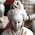Sunday, August 5, 2018

JCER Championship 2018, Match Komodo 9.02 - Fire 7.1

P
Engine Score 123456789012345678901234 +/-/=
1: Fire 7.1 14.0 / 24 =1=1=1=0========1=01==1= (+6 -2 =16)
2: Komodo 9.02 10.0 / 24 =0=0=0=1========0=10==0= (+2 -6 =16)

Temp 3' + 3" Hardware: Intel Core i7-4710MQ 7,9 GB Mem , 24 Tournament games,
GUI-Arena 3.5.1,
97.598 JCER games (01.07.2018) Book: Perfect 2017, Table created - Scid vs PC

1.1.